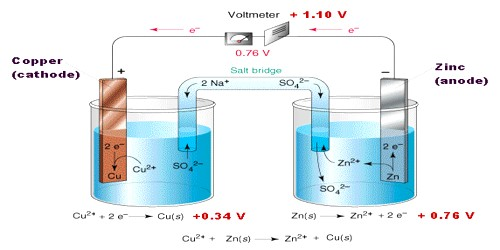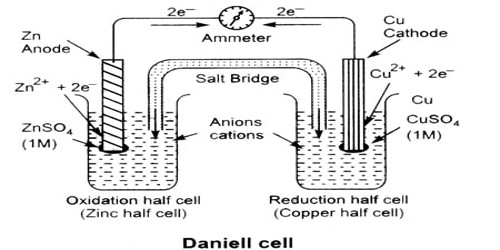Chemistry

# Galvanic CellsGalvanic Cells

An electrochemical cell is an arrangement in which electric energy is released by a spontaneous redox reaction. This kind of cell is also known as Galvanic cell or Voltaic cell named after two Italian scientists Luigi Galvani (1780) and Alessandro Volta (1800) who first conducted several experiments on chemical reactions and electric current. A common example of such a cell is Daniel Cell. A schematic diagram of the Daniel cell is given in Figure.Fig: Schematic diagram of Daniel cell

The cell essentially consists of a zinc rod dipped into a solution of zinc sulphate and copper rod dipped into a solution of copper sulphate, the two solutions being separated by a porous partition which allows the solutions to come in contact with each other but prevents complete mixing from taking place. The electrodes are metal wires/rods/sheets/bars which are dipped into the solutions. When the two electrodes (zinc and copper rods) are connected externally by a wire, electric current flows (as shown by the ammeter). Zinc dissolves from the zinc rod giving zinc ions whereas copper ions deposit on the topper rod as metallic copper. A voltmeter (not shown in the Figure) placed in the circuit measures the potential difference between the two electrodes. This is the electro motive force (e.m.f) of the cell.

The reactions taking place in a Daniel cell can be split up into two parts taking place at the two electrodes, each reaction being known as a half-reaction. At the zinc electrode oxidation takes place. So it is the anode: electrons released at this electrode move through the wire as indicated in Figure to the copper electrode ( which is the cathode) where these combine with copper ions to produce copper by reduction. As long as the electrodes are connected by a wire, electrons will be released at the anode and flow to the cathode (current will pass) until either zinc rod or copper ions are exhausted. Equations for the two half — reactions are shown below:

Zinc electrode (anode):    Zn(s) → Zn2+ (aq) + 2e … … … (1)

Copper electrode (cathode): Cu2+ (aq) +2e → Cu (s) … … … (2)

The sum of reactions (1) and (2) gives the complete cell reaction:

Zn (s) + Cu2+ (aq) → Zn2+ (aq) + Cu (s).